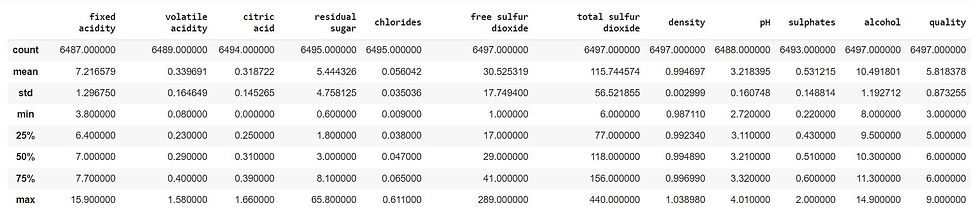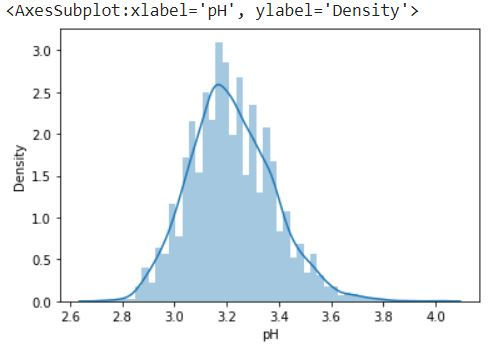top of page
• Hackers Realm

# Standardize data using Z-Score/Standard Scalar | Python

Standardization, often referred to as Z-score normalization or standard scaling, is a data preprocessing technique that plays a pivotal role in making data suitable for various analytical processes. Standardize data using standard scalar involves transforming the original data where the mean is set to zero and the standard deviation is equal to one.Standardize data using Z-Score/Standard Scalar

The methodology for standardization typically involves subtracting the mean of the dataset from each data point and dividing the result by the standard deviation. This operation transforms the data into a distribution with a mean of zero and a standard deviation of one, creating a standard Z-Score for each data point. The result is a set of values that can be directly compared, interpreted, and used in various statistical tests and machine learning algorithms.

You can watch the video-based tutorial with step by step explanation down below.

## Import Modules

```import pandas as pd
import seaborn as sns
import matplotlib.pyplot as plt
import warnings
import numpy as np
warnings.filterwarnings('ignore')
%matplotlib inline```
• pandas - used to perform data manipulation and analysis.

• seaborn - provides a high-level interface for creating attractive and informative statistical graphics. Seaborn is particularly useful for visualizing relationships between variables, exploring distributions, and presenting complex statistical analyses.

• matplotlib.pyplot - used for data visualization and graphical plotting.

• warnings - used to control and suppress warning messages that may be generated by the Python interpreter or third-party libraries during the execution of a Python program.

• numpy - used to perform a wide variety of mathematical operations on arrays.

## Import Data

Next we will read the data from the csv file .

```df = pd.read_csv('data/winequality.csv')First 5 rows of the dataframe
• The code snippet reads a CSV file named 'winequality.csv' into a Pandas DataFrame object named 'df' and then displaying the first few rows of the DataFrame using the head() function.

Next we will see the statistical summary of the DataFrame.

`df.describe()`summary of the dataframe
• The describe() function in Pandas provides a statistical summary of the DataFrame, including various descriptive statistics such as count, mean, standard deviation, minimum value, 25th percentile (Q1), median (50th percentile or Q2), 75th percentile (Q3), and maximum value for each numerical column in the DataFrame.

In this tutorial, we will be using fixed_acidity and pH columns for demonstrating the standardization of data using z-score/Standard Scaler.

## Visualize the Data

Next we will plot fixed acidity column.

`sns.distplot(df['fixed acidity'])`Distribution plot of fixed acidity
• The above code snippet creates a distribution plot for the 'fixed acidity' column in a DataFrame using Seaborn.

Next we will plot another column.

`sns.distplot(df['pH'])`Distribution plot of pH
• The above code snippet creates a distribution plot for the 'pH' column in a DataFrame using Seaborn.

## Standardization using Z-Score method

First we will create a copy of the dataframe.

`scaled_data = df.copy()`
• df.copy() is a method in pandas that creates a copy of the DataFrame. This copy is independent of the original DataFrame. In other words, any modifications you make to scaled_data will not affect the original df, and vice versa. It's a way to work with a duplicate of your data without altering the original data.

Next we will calculate the z-score.

```for col in ['fixed acidity', 'pH']:
scaled_data[col] = (scaled_data[col] - scaled_data[col].mean()) / scaled_data[col].std()

```
• for loop iterates through the list of column names 'fixed acidity' and 'pH'.

• for each column specified in the loop, we standardize the values in that column.

• scaled_data[col] refers to the specific column you are working with in the current iteration of the loop.

• scaled_data[col].mean() calculates the mean (average) of the values in that column.

• scaled_data[col].std() calculates the standard deviation of the values in that column.

• (scaled_data[col] - scaled_data[col].mean()) / scaled_data[col].std() subtracts the mean from each value in the column and then divides the result by the standard deviation. This operation scales the values to have a mean of 0 and a standard deviation of 1, which is the essence of standardization.

Next we will plot fixed_acidity column.

`sns.distplot(scaled_data['fixed acidity'])`Distribution plot of fixed_acidity column after standardization
• Before standardization the deviation of fixed acidity column was in the range of 4 to 16. Now we can see the standard deviation is in the range of -2 to +2.

Next we will plot pH column.

`sns.distplot(scaled_data['pH'])`Distribution plot of pH column after standardization
• Before standardization the deviation for pH column was in the range of 2.8 to 3.8. Now we can see the standard deviation is in the range of -2 to +2.

## Standardization using StandardScaler

Previously we did manual standardization using formula, now we will see how we can use StandardScaler library for standardization.

First we will import the module.

```from sklearn.preprocessing import StandardScaler
sc = StandardScaler()```
• We import the StandardScaler class from scikit-learn.

• Next we create an instance of the StandardScaler class, named sc.

Next we will train the data.

`sc.fit(df[['pH']])`

StandardScaler()

• sc.fit(df[['pH']]) is using the fit method of the StandardScaler object (sc) to compute the mean and standard deviation of the 'pH' column in your original DataFrame df.

• This step is necessary to determine the parameters for scaling the data properly.

Next we will standardize or scale data.

`sc_data = sc.transform(df[['pH']])`
• This code applies the scaling transformation to the 'pH' column in the DataFrame df using the parameters (mean and standard deviation) that were calculated when you called sc.fit(df[['pH']]). The transform method scales the data based on those parameters.

• The scaled data is then assigned to the variable sc_data. This variable will contain the 'pH' column after it has been standardized.

Next we will reshape the output.

`sc_data = sc_data.reshape(-1)`
• This code reshapes the sc_data array into a one-dimensional array. The -1 argument in the reshape function is used to infer the size of one dimension while keeping the total number of elements the same. In this case, it effectively flattens the array into a 1D shape.

Next we will plot the pH column before standardization.

`sns.distplot(df['pH'])`Distribution plot of pH column before standardization

Next we will plot the pH column after standardization.

`sns.distplot(sc_data)`Distribution plot of pH column after standardization
• We can see that we have got the same result as Z-Score method by using StandardScaler method as well.

## Final Thoughts

• Standardization is crucial for ensuring that features contribute equally to the modeling process.

• It helps prevent features with large values from dominating the modeling process.

• While it's a common practice, it's essential to consider the specific requirements of your analysis or machine learning task. In some cases, you might choose not to standardize data if it doesn't provide any benefits.

In summary, data preprocessing, including standardization, depends on the nature of your data and the requirements of your analysis or modeling task. It's often a good practice to experiment with both standardized and non-standardized data to see which approach works best for your specific use case.

Get the project notebook from here